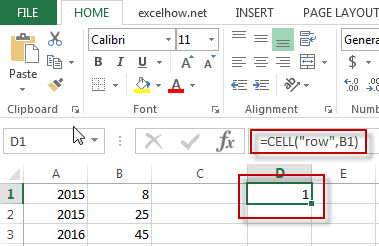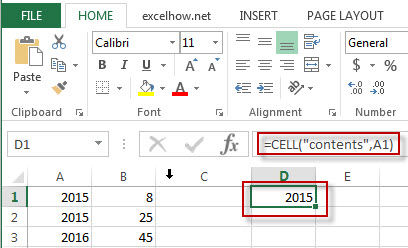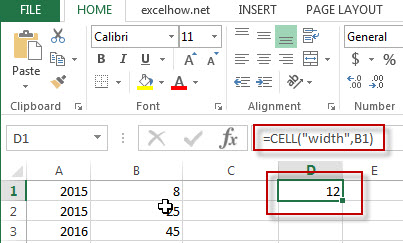# Excel Cell Function

This post will guide you how to use Excel CELL function with syntax and examples in Microsoft excel.

### Description

The Excel CELL function returns information about the formatting, location, size, or contents of a cell.

The CELL function is a build-in function in Microsoft Excel and it is categorized as an Information Function.

The CELL function is available in Excel 2016, Excel 2013, Excel 2010, Excel 2007, Excel 2003, Excel XP, Excel 2000, Excel 2011 for Mac.

### Syntax

The syntax of the CELL function is as below:

= CELL (info_type,[reference])

Where the CELL function arguments are:
Info_type -This is a required argument. The type of information that you want to get. The following types can be used.

From Microsoft site:

 info_type Returns “address” Reference of the first cell in reference, as text. “col” Column number of the cell in reference. “color” The value 1 if the cell is formatted in color for negative values; otherwise returns 0 (zero). NOTE: This value is not supported in Excel Online, Excel Mobile, and Excel Starter. “contents” Value of the upper-left cell in reference; not a formula. “filename” Filename (including full path) of the file that contains reference, as text. Returns empty text (“”) if the worksheet that contains reference has not yet been saved. NOTE: This value is not supported in Excel Online, Excel Mobile, and Excel Starter. “format” Text value corresponding to the number format of the cell. The text values for the various formats are shown in the following table. Returns “-” at the end of the text value if the cell is formatted in color for negative values. Returns “()” at the end of the text value if the cell is formatted with parentheses for positive or all values. NOTE: This value is not supported in Excel Online, Excel Mobile, and Excel Starter. “parentheses” The value 1 if the cell is formatted with parentheses for positive or all values; otherwise returns 0. NOTE: This value is not supported in Excel Online, Excel Mobile, and Excel Starter. “prefix” Text value corresponding to the “label prefix” of the cell. Returns single quotation mark (‘) if the cell contains left-aligned text, double quotation mark (“) if the cell contains right-aligned text, caret (^) if the cell contains centered text, backslash (\) if the cell contains fill-aligned text, and empty text (“”) if the cell contains anything else. NOTE: This value is not supported in Excel Online, Excel Mobile, and Excel Starter. “protect” The value 0 if the cell is not locked; otherwise returns 1 if the cell is locked. NOTE: This value is not supported in Excel Online, Excel Mobile, and Excel Starter. “row” Row number of the cell in reference. “type” Text value corresponding to the type of data in the cell. Returns “b” for blank if the cell is empty, “l” for label if the cell contains a text constant, and “v” for value if the cell contains anything else. “width” Column width of the cell, rounded off to an integer. Each unit of column width is equal to the width of one character in the default font size. NOTE: This value is not supported in Excel Online, Excel Mobile, and Excel Starter.

### Example

The below examples will show you how to use Excel CELL Function to retrieve information about a cell.

#1 =CELL(“row”,B1)Note: the above excel formula will return the row number of Cell B1.

#2 =CELL(“contents”,A1)Note: The above excel formula will return the content of cell A1.

#3 =CELL(“width”,B1)Note: the above excel formula will return the column width of the cell B1.

### More CELL Function Examples

• Quickly Get Sheet Name
If you want to quickly get current worksheet name only, then inert it into one cell. You can use a formula based on the MID function in combination with the FIND function and the Cell function……
• Insert The File Path and Filename into Cell
If you want to insert a file path and filename into a cell in your current worksheet, you can use the CELL function to create a formual……..